## Prediction Comparison of Flow Resistance in Channels with Rounded and Angular Coarse Rough Beds 111

Citation: Prediction Comparison of Flow Resistance in Channels with Rounded and Angular Coarse Rough Beds, American Research Journal of Civil and Structural Engineering, vol 1, no. 1, pp. 1-15.

Copyright This is an open access article distributed under the Creative Commons Attribution License, which permits unrestricted use, distribution, and reproduction in any medium, provided the original work is properly cited.

## Abstract:

Manning’s (n) is a coefficient representing quantity of friction which a channel applies against flow. In general, it explains how roughness is a channel. This experimental research tries to explore (n) by making 8 m length, 40 cm width and 40 cm height laboratory flume with adjustable slope. The flume was used to carry out a total of 72 experiments with 4 different slopes, 3 different flow rates and 2 types of sorting with angular and rounded aggregates. The results showed that the roughness coefficient of the beds covered with angular grains is on average 6.68% higher than that of the beds covered with rounded grains. Also, for a constant value, if the flow rate increases, Manning roughness coefficient will also decrease. It was also shown that parameters such as relative submergence, grain size and slope affect the roughness coefficient. Based on the results of this research, it can be concluded that in all of the experiments, the shear stress of the bed with the rounded particles declined 3.62% as compared to angular particles.

Keywords: Manning roughness coefficient, angular, rounded, rough bed

## Description:

INTRODUCTION

So far, many studies have been performed to estimate the roughness coefficient of flow resistance due to the size of the particles, but in these studies, the effect of particle shape is not clearly understood. In alluvial channels of Paul and Jarvis (1976), Bathurst and Ferguson (1986), Petit (1990), San Verida (1991), Robert et al (1992), Prandtl-Karman principle and vertical velocity distribution were used that velocity can be dependent on the logarithm of height. Shear velocity can be calculated according to velocity distribution equations.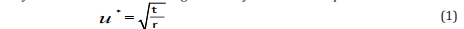where u* is shear velocity, τ is shear stress and ρ is water density. In totally rough flows, local (spatial) estimation of shear velocity determines the dynamics of sedimentation which may seriously deviate from the results of equation (2). It is due to the significant variability of bed roughness.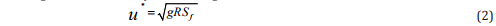where u* is shear velocity, g is gravity, R is hydraulic radius and Sf is the energy line slope.
Some literatures studying the effect of sediment particle sizes of the bed and the waterway walls on Manning roughness coefficient (n) are: Strickler (1923), Meyer-Peter and Mueller (1948), Keulegan (1938), Henderson (1966), Anderson et.al (1970) and Huger (1970). They suggested that Manning roughness coefficient is just a function of average bed particle size. Strickler (1923) was the first to present the following equation for determining Manning roughness coefficient (n) based on his experimental observations about the rivers with eroded rocky beds in Switzerland: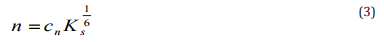where Cn is a factor dependent on the definition of Ks measurement unit, the type of the material covering the floor and walls of the channel. In rivers and sedimentary channels where the bed is made of non-cohesive materials (sand, gravel, pebbles, etc.), the diameter of the particles forming the channel wall (D) can be used instead of Ks (Mahmoodian Shooshtari, 2006). On the effect of slope on Manning roughness coefficient, Hessel, Jeten and Guanxo (2003) conducted some experiments to estimate Manning roughness coefficient for the steep slopes. Jarrett (1984) proposed the following equation to estimate the Manning coefficient: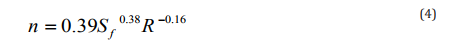where Sf is the slope of the energy line and R is the hydraulic radius in feet. Hydraulically, the flow can be steady, transient or rough. Hydraulically, a steady flow occurs when surficial disturbances are so small that all the roughness elements are generally immersed below the linear layer (Chow, 1959).
Graf (1998), Schlichting and Gersten (2000) defined the flow with smooth, transient and rough beds as follows: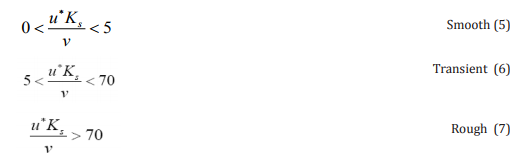where u* is the shear velocity and k is the roughness height. Thus, velocity distribution is affected by bed roughness and viscosity (Chow, 1959).

MATERIALS AND METHODS

According to the research objectives, the experiments were conducted using a flume located at Hydraulic Laboratory of Shahrekord University. The flume was rectangular with adjustable slope (length, width and height of 8 m, 40 cm and 40 cm, respectively). To perform the tests, both particle shapes (angular and rounded) were provided in three average grain sizes (10, 20 and 30 mm). Then, for each particle shape, the flume bed was uniformly covered with the intended grading between its both ends with a height that was at least twice the height of D50. Then by creating a uniform flow through the gate located at the end of the flume, water depth readings according to the 4 tested slopes of 0.005, 0.01, 0.015 and 0.02, and the three (initial, intermediate, and final) flow rates of 10, 20 and 30 liters per second, three sections were considered along the flume at a spacing of 2 m. Also, the velocity at every section was measured using pitot tube at 0.2, 0.6 and 0.8 of the water depth at three points of the width of the flume. In order to roughen the river bed by using of sediments, three different gradations in 10, 20 and 30 millimeter sizes were used. Rounded sediments (collected from the river) and angular sediments (produced from the crusher) were employed after they were prepared and graded.

Figure1,2 show an example of angular and rounded particles with 2 gradations, respectively.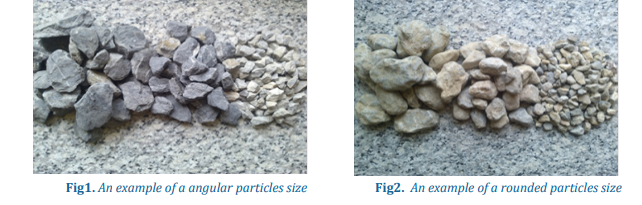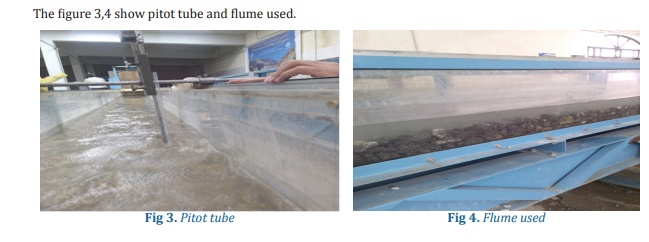The factors affecting Manning roughness coefficient of rough beds are: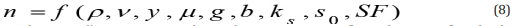where n is Manning roughness coefficient, ρ is unit volume of water, v is average flow velocity, y is flow depth, μ is mechanical viscosity coefficient of water, g is gravity, and b is the width of the channel that was constant in this study (b= 0.4m). ks is the average size of the bed particles, s0 is the slope of channel floor, and SF is the shape factor of sedimentary aggregates.

Using Buckingham’s relation and by selecting the three variables of ρ, v and y as the repetitive variables, equation 4 can be transformed to equation 5 that includes 5 parameters: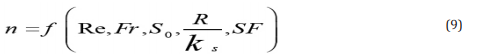where Re is the Reynolds number, Fr is the Froude number of flow, S0 is the channel bottom slope, is the parameter of relative submergence and SF is the shape factor of the aggregates.

RESULTS AND DISCUSSION

The effect of relative submergence on Manning roughness coefficient

In order to investigate the effect of the shape of sedimentary particles on Manning roughness coefficient, the (n) diagram was plotted versus k/ks in Fig. 5 for different flow rates for the bed sediments with the same grading.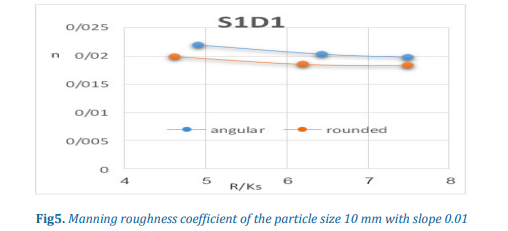As the graph shows, In the same hydraulic conditions, the flow lines are more easily separated from the surface of the angular particles compared to rounded particles, i.e. the separation points of angular particles occur before that of the rounded ones. Thus, the detachment zone created behind every angular particle is larger than that of a rounded particle. As a result, the pressure difference between the front and behind the angular particles is more than that of the rounded particles. As a result, on a rough bed, the total drag forces for angular particles are higher than that of the rounded particles; in another word, the frictional loss of angular particles is higher than that of rounded particles which is consistent with the findings of the study by Shafaei Bajestan (2010).

THE EFFECT OF GRAINS SIZE ON MANNING ROUGHNESS COEFFICIENT

Along a rough bed in the flow direction, both the frictional drag force and the pressure drag of the fluid affect the bed. At the same hydraulic condition, the greater the roughness of the bed, the higher the drag force, since the grain surfaces facing the flow is increased. Therefore, at the same hydraulic conditions, if the particle size increases, Manning roughness coefficient increases. As a result, the drag force becomes smaller which results in reduced drag pressure. Therefore, increased flow rate and depth lead to decreased total drag force, and thereby, decreases the Manning roughness coefficient (Eslamian S. and et al. 2017 & Shayannejad M. and et al. 2015 & Ostad-Ali-Askari and et al. 2015).

As the diagrams in Fig. 6 shows, by increasing the particle size, the Manning roughness coefficient increases; and the increase in roughness coefficient of angular grains is much higher than that of the rounded grains.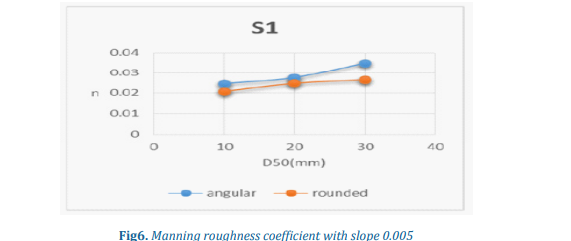The table 1 shows Using SPSS, and with regard to Manning roughness coefficient (n) as the dependent variable, R/Ks , S0 , and D as independent variables, linear regression analysis was carried out on both rounded and angular beds.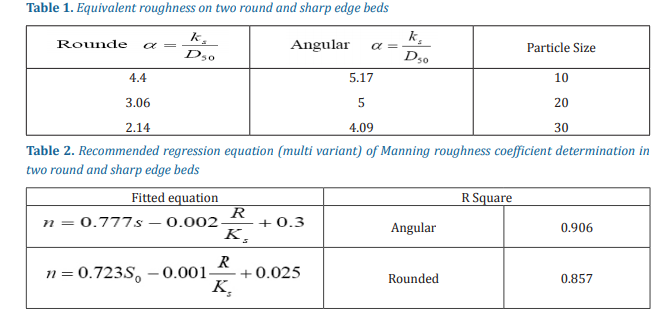The table 2 shows This type of regression is a method for finding the relationship between the variable and a set of independent variables.
The equal roughness of the rivers is usually considered equal to a coefficient that is representative of the sedimentary grains of the bed,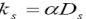The value of α in the rivers with uniform bed materials is equivalent to 1 and D50 is assumed as the representative size of the bed materials.
As is clear from the table, the larger the particle, the less the equivalent roughness. For angular beds, the value of this coefficient is higher.
Comparison of the velocity distribution for both rounded and angular cases
The flow with rough bed occurs when the roughness is 6 times δ. In these types of flows, the shear Reynolds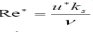is also greater than 70. These types of flows are also called fully turbulent flows.
As diagram in Fig. 6 shows, the velocity near water surface is greater than the bottom depth of the bed which is due to the existence of secondary flows and fineness ratio.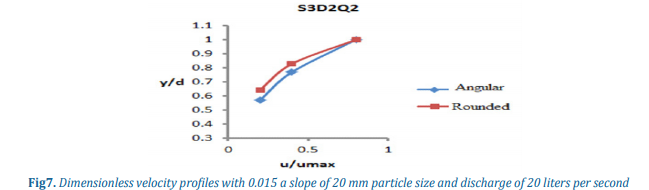Comparison of shear stress distribution of the bed for both rounded and angular cases
As the curve in Fig. 7 shows, the bed shear stress increased with increasing bed particle sizes and the shear stress of angular bed is more than the rounded bed. At the same hydraulic conditions, the flow lines are separated easier from the surfaces of angular particles than that of the rounded particles, i.e. the separation points of angular particles occur before that of the rounded aggregates. Consequently, the pressure difference between the two sides of angular particles is higher than that of the rounded particle. This causes the drag pressure of angular particles to be greater than that of the rounded particles. As a result, the total drag force acting on the rough bed for angular aggregates is higher than the rounded particles, or in other words, the friction losses of angular particles is more than that of the rounded particles. This causes the shear stress of angular particles to be higher than that of the rounded particles.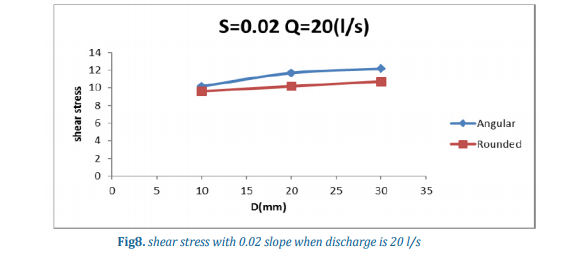DIMENSIONLESS VELOCITY DISTRIBUTION; ANGULAR AND ROUNDED

The dimensionless images of velocity distribution in Fig. 9 and 10 suggest that in the rounded bed the slope of velocity variations is milder than the slope of changes in the angular bed. These conditions resemble those of old and young rivers: the angular bed resembles the young rivers whereas the rounded bed is similar to the old rivers.